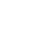## 抽象语法树是什么

### 抽象语法树举例

``````foo = 'hello world';
/*
+-------------+
|  assign(=)  |
+-------------+
X        X
X          X
+-------+    +-----------------+
|  foo  |    |  'hello world'  |
+-------+    +-----------------+
*/
``````
``````if (foo === true) {
bar = 'hello world';
}
/*
+------+
|  if  |
+------+
X    X
X        X
+--------------+    +-------------+
|  equal(===)  |    |  if_body    |
+--------------+    +-------------+
X        X              X         X
X         X                X          X
+-------+   +--------+    +-------------+   +------------+
|  foo  |   |  true  |    |  assign(=)  |   |  alert()   |
+-------+   +--------+    +-------------+   +------------+
X        X                  X
X            X                  X
+-------+   +-----------------+    +-------+
|  bar  |   |  'hello world'  |    |  bar  |
+-------+   +-----------------+    +-------+
*/
``````

## JavaScript 抽象语法树

### UglifyJS

UglifyJS 是使用最广的 JavaScript 压缩工具之一，而且自身也是用 JavaScript 写的，使用它的方法很简单（需要 nodejs 环境）：

``````[sudo ]npm install -g uglify-js
``````

``````uglifyjs -m srcFileName.js -o destFileName.min.js
``````

### UglifyJS Tools

UglifyJS 提供了一些工具用于分析 JavaScript 代码，包括：

• parser，把 JavaScript 代码解析成抽象语法树
• code generator，通过抽象语法树生成代码
• mangler，混淆 JavaScript 代码
• scope analyzer，分析变量定义的工具
• tree walker，遍历树节点
• tree transformer，改变树节点

### 生成抽象语法树

``````npm install uglify-js --save-dev
``````

``````var UglifyJS = require('uglify-js');

var ast = UglifyJS.parse('function sum(foo, bar){ return foo + bar; }');
``````

### 使用 mangler 压缩代码

``````var UglifyJS = require('uglify-js');

var ast = UglifyJS.parse('function sum(foo, bar){ return foo + bar; }');
ast.figure_out_scope();
ast.mangle_names();
console.log(ast.print_to_string());
// function sum(a,b){return a+b}
``````

### 使用 walker 遍历抽象语法树

``````var UglifyJS = require('uglify-js');

var ast = UglifyJS.parse('function sum(foo, bar){ return foo + bar; }');
ast.figure_out_scope();
ast.walk(new UglifyJS.TreeWalker(function(node) {
console.log(node.print_to_string());
}));
/*
function sum(foo,bar){return foo+bar}
function sum(foo,bar){return foo+bar}
sum
foo
bar
return foo+bar
foo+bar
foo
bar
*/
``````

UglifyJS 已经提供了直接压缩代码的脚本，walker 看上去貌似也没啥用，那么这些工具有什么使用场景呢？

## 抽象语法树的应用

### 利用抽象语法树重构 JavaScript 代码

``````parseInt('10.23');     // 10            转换成正整数
parseInt('10abc');     // 10            忽略其他字符
parseInt('10', 10);    // 10            转换成十进制
parseInt('10', 2);     // 2             转换成二进制
parseInt('0123');      // 83 or 123     不同浏览器不一样，低版本浏览器会转换成八进制
parseInt('0x11');      // 17            转换成十六进制
``````

``````#! /usr/bin/env node

var U2 = require("uglify-js");

function replace_parseint(code) {
var ast = U2.parse(code);
// accumulate `parseInt()` nodes in this array
var parseint_nodes = [];
ast.walk(new U2.TreeWalker(function(node){
if (node instanceof U2.AST_Call
&& node.expression.print_to_string() === 'parseInt'
&& node.args.length === 1) {
parseint_nodes.push(node);
}
}));
// now go through the nodes backwards and replace code
for (var i = parseint_nodes.length; --i >= 0;) {
var node = parseint_nodes[i];
var start_pos = node.start.pos;
var end_pos = node.end.endpos;
node.args.push(new U2.AST_Number({
value: 10
}));
var replacement = node.print_to_string({ beautify: true });
code = splice_string(code, start_pos, end_pos, replacement);
}
return code;
}

function splice_string(str, begin, end, replacement) {
return str.substr(0, begin) + replacement + str.substr(end);
}

// test it

function test() {
if (foo) {
parseInt('12342');
}
parseInt('0012', 3);
}

console.log(replace_parseint(test.toString()));

/*
function test() {
if (foo) {
parseInt("12342", 10);
}
parseInt('0012', 3);
}
*/
``````

``````var foo = 'parseInt("12345")';
// parseInt("12345");
``````

### 抽象语法树在美团中的应用

``````YUI.add('mod1', function(Y) {
Y.one('#button1').simulate('click');
Y.Array.each(array, fn);
Y.mod1 = function() {/**/};
}, '', {
requires: [
'node',
'array-extras'
]
});
Y.mod1();
// Y.io(uri, config);
}, '', {
requires: [
'mod1',
'io'
]
});
``````

``````YUI.add('mod1', function(Y) {
Y.one('#button1').simulate('click');
Y.Array.each(array, fn);
Y.mod1 = function() {/**/};
}, '', {
requires: [
'node',
'node-event-simulate'
]
});
Y.mod1();
// Y.io(uri, config);
}, '', {
requires: [
'mod1'
]
});
``````

1. 找到代码中模块定义（`YUI.add`）的部分
2. 分析每个模块内函数定义，变量定义，赋值语句等，找出符合要求（以 `Y` 开头）的输出接口（如 `mod1` 中的 `Y.mod1`
3. 生成「接口 - 模块」对应关系
4. 分析每个模块内函数调用，变量使用等，找出符合要求的输入接口（如 `mod2` 中的 `Y.one``Y.Array.each``Y.mod1`
5. 通过「接口 - 模块」对应关系，找到此模块应该依赖哪些其他模块
6. 分析 requires 中是否有错误

## Reference